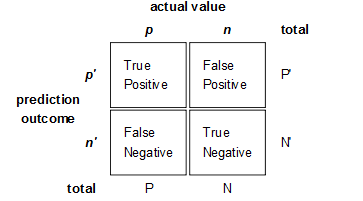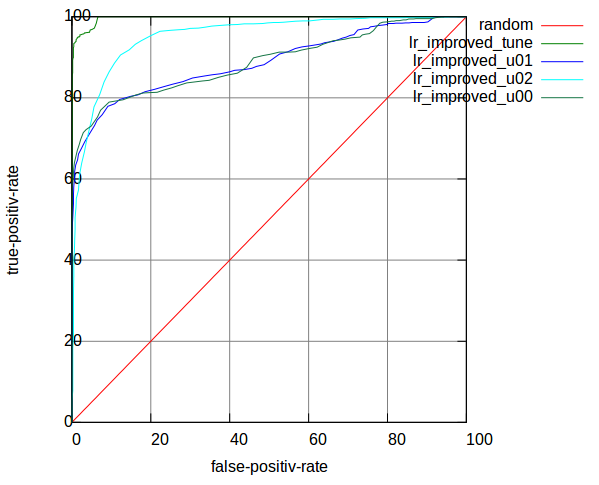Spam Filter (1) -- Data Resource & ROC Curve

This is my project of CS559 Machine Learning @Stevens 2013 Fall.

This project will design a Spam Filter based on the date set from Discovery Challenge workshop at ECML/PKDD 2006 in Berlin.

Abstract

• I used two basic machine learning technologies learned from the class: Naive Bayes and Logistic Regression.
• And also made some improvement based on practical implementation.
• Another improvement I have used is Self-Learning which augments the evaluation data on training set.

Introduction

This competition was held at German in 2006. I finished the Task A in this challenge. The evaluation criterion is the AUC value which I will explain a bit of it later on. The first ranked team has accessed 0.95 Average AUC during the challenge and up to 0.98 updated after the challenge. I get the Average AUC above 0.95, too.

Experiment Data

Give an example of a mail, this is the first line of the first mail in task_labeled_train.tf:

1 9:3 94:1 109:1 163:1

This line represents a spam email (starting with class label “1”) with four words. The word ID of the first token is 9 and this word occurs 3 times within this email, indicated by “:3”.

AUC & ROC

ROC (Receiver Operating Characteristic) is a graphical plot which illustrates the performance of a binary classifier system as its discrimination threshold is varied. It is created by plotting the fraction of true positives out of the total actual positives (TPR = true positive rate) vs. the fraction of false positives out of the total actual negatives (FPR = false positive rate), at various threshold settings.Figure 1: The four possible labels of predict result

Here gives a sample of ROC curves in Figure 2. Where X-axis, called Specificity, is False Positive rate = FP / [FP + TN]; Y-axis, called Sensitivity, is True Positive rate = TP / [TP + FN].Figure 2: Sample of ROC curves

AUC (Area Under Curve): The AUC value is the area under the ROC curve. The AUC value will between 0 and 1, higher value means a better performance.

The Two Types of Classifiers

There are two types of Machine Learning Techniques: Discriminative Model (Conditional Model) and Generative Model.

 Discriminative Model is modeling the conditional probability distribution P(y x), which can be used for predicting y from x. It includes Logistic Regression, SVM, etc.

Generative Model specifies a joint probability distribution over observation and label sequences. It includes Naive Bayes, etc.

I have used Naive Bayes (NB) and Logistic Regression (LR) in my project.

Author: Renjie Weng
Project repository: GiHub Pages
SlideShow
Formal report: PDF & PPT
 Tweet